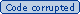# Norm

“In linear algebra, functional analysis and related areas of mathematics, a norm is a function which assigns a positive length or size to all vectors in a vector space, other than the zero vector.”
Wikipedia (2006)

“The norm of a mathematical object is a quantity that in some (possibly abstract) sense describes the length, size, or extent of the object.”
MathWorld (2006)